##### The Japanese Maple specialist
Direct order Contact Help / Services Newsletter# Shelf to displaySearch

Japanese MaplesYoung trees Acer seeds and more Bonsai soil and fertiliserVarieties introduced into EuropeHostasWind bells and chimesSaxifragesSpecial offer

Packing and shipping charges

# Hubbles super cork

› Japanese maples › Acer palmatum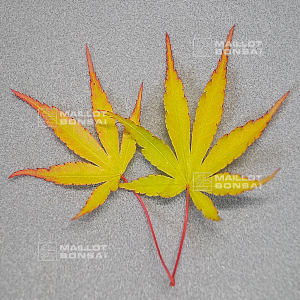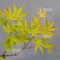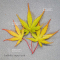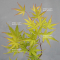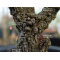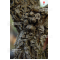ref. : 12001

Choose the right size
Pot size

This item is temporarily unavailable

###### Description

Observing at Maillot Bonsai for 5 years, the first signs of the corky appearance were noticed as early as the second year.
The "Super Cork" by Hubble is a new introduction of one of the finest selections of pin-bark maple by Scott Hubble of Metro Maples in Texas. This selection produces the corkiest bark among all rough-bark Japanese maple varieties to date, and does so at an earlier age. This gives 'Hubble's Super Cork' a more mature appearance from a young age. The foliage is green in summer and turns orange and then red in autumn. We expect 'Hubble's Super Cork' to reach 2 to 3 meters in 15 years. It thrives well in sunny conditions.

#super 5.5 #cork 5.4 #hubble 4.6 #bark 3.4 #appearance 3 #palmatum 2.8 #hubbles 2.7 #years 2.5 #maple 2.5 #acer 2.4

###### Technical description
 Species Acer palmatum same species items Variety Hubbles super cork Leaf colour Green leaves same colour items Leaf shape Palmated same shaped items Adult height Between 2 and 4 meters same height items Shape Natural same shape items Exposure Sun same exposure items

Formule
(( ROUND((CHAR_LENGTH(b.article_nom)-CHAR_LENGTH(REPLACE(b.article_nom, 'super', '')))/LENGTH('super')) + ROUND((CHAR_LENGTH(b.article_description)-CHAR_LENGTH(REPLACE(b.article_description, 'super', '')))/LENGTH('super')) ) * 5.5) + (( ROUND((CHAR_LENGTH(b.article_nom)-CHAR_LENGTH(REPLACE(b.article_nom, 'cork', '')))/LENGTH('cork')) + ROUND((CHAR_LENGTH(b.article_description)-CHAR_LENGTH(REPLACE(b.article_description, 'cork', '')))/LENGTH('cork')) ) * 5.4) + (( ROUND((CHAR_LENGTH(b.article_nom)-CHAR_LENGTH(REPLACE(b.article_nom, 'hubble', '')))/LENGTH('hubble')) + ROUND((CHAR_LENGTH(b.article_description)-CHAR_LENGTH(REPLACE(b.article_description, 'hubble', '')))/LENGTH('hubble')) ) * 4.6) + (( ROUND((CHAR_LENGTH(b.article_nom)-CHAR_LENGTH(REPLACE(b.article_nom, 'bark', '')))/LENGTH('bark')) + ROUND((CHAR_LENGTH(b.article_description)-CHAR_LENGTH(REPLACE(b.article_description, 'bark', '')))/LENGTH('bark')) ) * 3.4) + (( ROUND((CHAR_LENGTH(b.article_nom)-CHAR_LENGTH(REPLACE(b.article_nom, 'appearance', '')))/LENGTH('appearance')) + ROUND((CHAR_LENGTH(b.article_description)-CHAR_LENGTH(REPLACE(b.article_description, 'appearance', '')))/LENGTH('appearance')) ) * 3) + (( ROUND((CHAR_LENGTH(b.article_nom)-CHAR_LENGTH(REPLACE(b.article_nom, 'hubbles', '')))/LENGTH('hubbles')) + ROUND((CHAR_LENGTH(b.article_description)-CHAR_LENGTH(REPLACE(b.article_description, 'hubbles', '')))/LENGTH('hubbles')) ) * 2.7) + (( ROUND((CHAR_LENGTH(b.article_nom)-CHAR_LENGTH(REPLACE(b.article_nom, 'years', '')))/LENGTH('years')) + ROUND((CHAR_LENGTH(b.article_description)-CHAR_LENGTH(REPLACE(b.article_description, 'years', '')))/LENGTH('years')) ) * 2.5) + (( ROUND((CHAR_LENGTH(b.article_nom)-CHAR_LENGTH(REPLACE(b.article_nom, 'maple', '')))/LENGTH('maple')) + ROUND((CHAR_LENGTH(b.article_description)-CHAR_LENGTH(REPLACE(b.article_description, 'maple', '')))/LENGTH('maple')) ) * 2.5) + (( ROUND((CHAR_LENGTH(b.article_nom)-CHAR_LENGTH(REPLACE(b.article_nom, 'this', '')))/LENGTH('this')) + ROUND((CHAR_LENGTH(b.article_description)-CHAR_LENGTH(REPLACE(b.article_description, 'this', '')))/LENGTH('this')) ) * 2.4) + (( ROUND((CHAR_LENGTH(b.article_nom)-CHAR_LENGTH(REPLACE(b.article_nom, 'introduction', '')))/LENGTH('introduction')) + ROUND((CHAR_LENGTH(b.article_description)-CHAR_LENGTH(REPLACE(b.article_description, 'introduction', '')))/LENGTH('introduction')) ) * 2.2)

## Secure payment## Delivery

Our logistic partners :04 74 55 23 48
Pépinière MAILLOT-BONSAÏ
Le Bois Frazy
01990 RELEVANT - FRANCE
on appointment